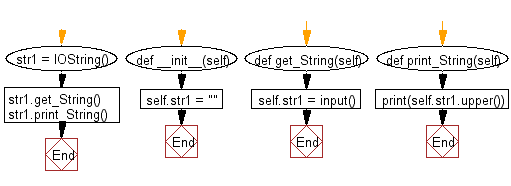﻿ Python: A class has two methods. First method accept a string from the user, Second method print the string in upper case - w3resource# Python: A class has two methods. First method accept a string from the user, Second method print the string in upper case

## Python Class: Exercise-9 with Solution

Write a Python class which has two methods get_String and print_String. get_String accept a string from the user and print_String print the string in upper case.

Sample Solution:

Python Code:

``````class IOString():
def __init__(self):
self.str1 = ""

def get_String(self):
self.str1 = input()

def print_String(self):
print(self.str1.upper())

str1 = IOString()
str1.get_String()
str1.print_String()
```
```

Sample Output:

```w3resource
W3RESOURCE
```

Pictorial Presentation:Flowchart:## Visualize Python code execution:

The following tool visualize what the computer is doing step-by-step as it executes the said program:

Python Code Editor:

What is the difficulty level of this exercise?

Test your Programming skills with w3resource's quiz.

﻿

## Python: Tips of the Day

The Zip() Function:

```>>> students = ('John', 'Mary', 'Mike')
>>> ages = (15, 17, 16)
>>> scores = (90, 88, 82, 17, 14)
>>> for student, age, score in zip(students, ages, scores):
...     print(f'{student}, age: {age}, score: {score}')
...
John, age: 15, score: 90
Mary, age: 17, score: 88
Mike, age: 16, score: 82
>>> zipped = zip(students, ages, scores)
>>> a, b, c = zip(*zipped)
>>> print(b)
(15, 17, 16)
```